# 基于 TensorFlow 2.0 的长短期记忆网络进行多类文本分类• 它们利用顺序信息。
• 它们具备记忆能力，能够记住到目前为止计算过的内容，也就是说，我最后说的内容将影响我接下来要讲的内容。
• 递归神经网络是文本和语音分析的理想选择。
• 最常用的递归神经网络是长短期记忆网络（Long-Short Term Memory，LSTM）。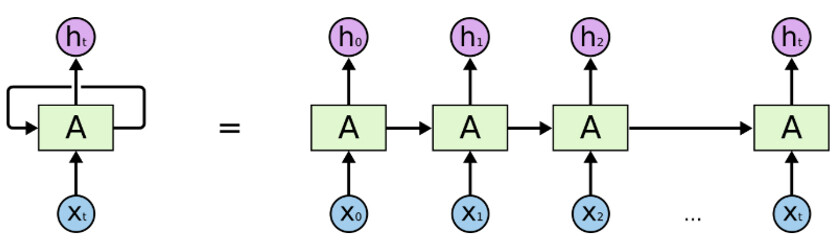• “A” 是前馈神经网络（Feedforward neural network）的一层。
• 如果我们只看右边的话，它会递归地遍历每个序列的元素。
• 如果我们将左边展开，它看起来将会跟右边一模一样。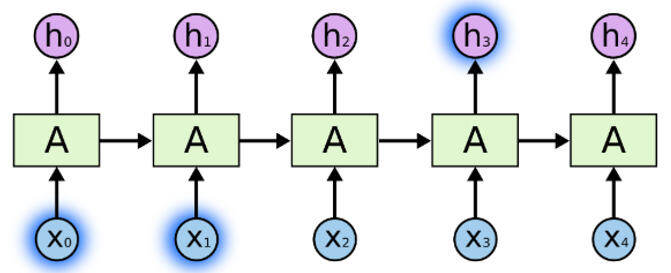• 我们输入每个单词，这些单词以某种方式相互关联。
• 当我们看到文章中所有的单词时，我们会在文章末尾做出预测。
• 递归神经网络通过传递上一次输出的输入，能够保留信息，并能够在最后利用所有信息进行预测。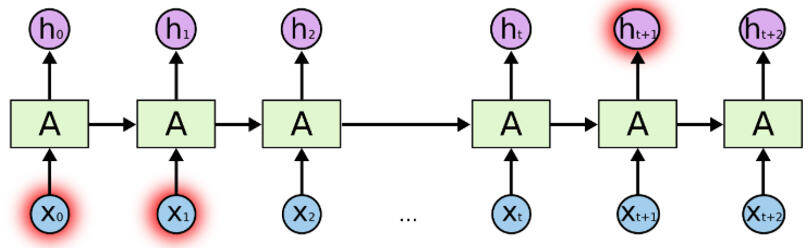• 这对于短句很有效，但当我们处理一篇长文章时，将会有一个长期依赖问题。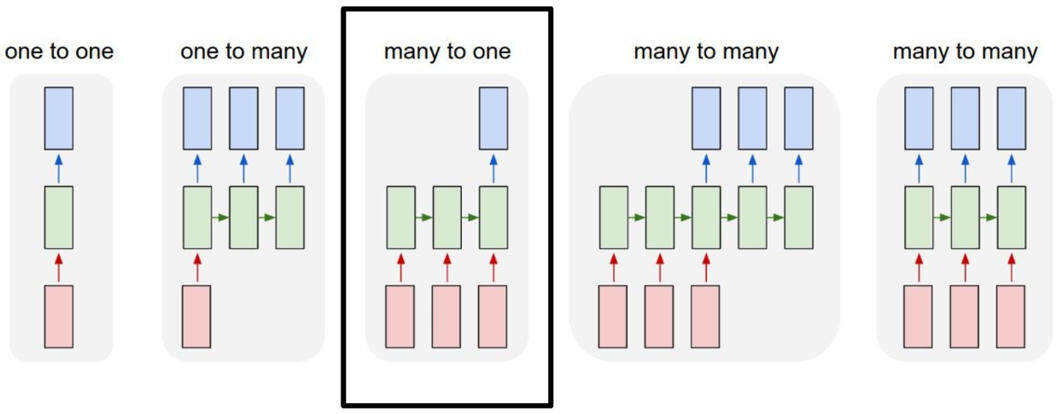• 首先，我们导入库，并确保 TensorFlow 是正确的版本。

import csvimport tensorflow as tfimport numpy as npfrom tensorflow.keras.preprocessing.text import Tokenizerfrom tensorflow.keras.preprocessing.sequence import pad_sequencesfrom nltk.corpus import stopwordsSTOPWORDS = set(stopwords.words('english'))print(tf.__version__)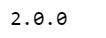• 将超参数置于顶部，如下所示，便于进行更改和编辑。
• 届时，我们将会讲解每个超参数是如何工作的。

vocab_size = 5000embedding_dim = 64max_length = 200trunc_type = 'post'padding_type = 'post'oov_tok = '<OOV>'training_portion = .8
• 定义两个包含文章和标签的列表。同时，我们删除了停用词。

articles = []labels = []with open("bbc-text.csv", 'r') as csvfile:    reader = csv.reader(csvfile, delimiter=',')    next(reader)    for row in reader:        labels.append(row)        article = row        for word in STOPWORDS:            token = ' ' + word + ' '            article = article.replace(token, ' ')            article = article.replace(' ', ' ')        articles.append(article)print(len(labels))print(len(articles))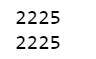train_size = int(len(articles) * training_portion)train_articles = articles[0: train_size]train_labels = labels[0: train_size]validation_articles = articles[train_size:]validation_labels = labels[train_size:]print(train_size)print(len(train_articles))print(len(train_labels))print(len(validation_articles))print(len(validation_labels))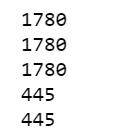tokenizer = Tokenizer(num_words = vocab_size, oov_token=oov_tok)tokenizer.fit_on_texts(train_articles)word_index = tokenizer.word_indexdict(list(word_index.items())[0:10])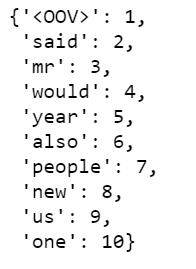train_sequences = tokenizer.texts_to_sequences(train_articles)print(train_sequences)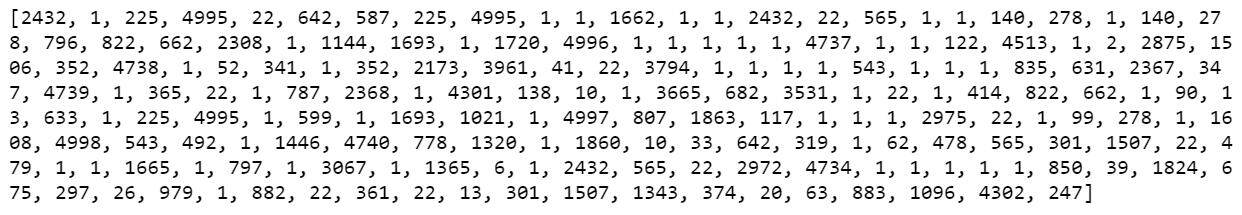" 图 1"

train_padded = pad_sequences(train_sequences, maxlen=max_length, padding=padding_type, truncating=trunc_type)print(len(train_sequences))print(len(train_padded))print(len(train_sequences))print(len(train_padded))print(len(train_sequences))print(len(train_padded))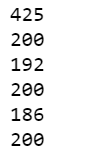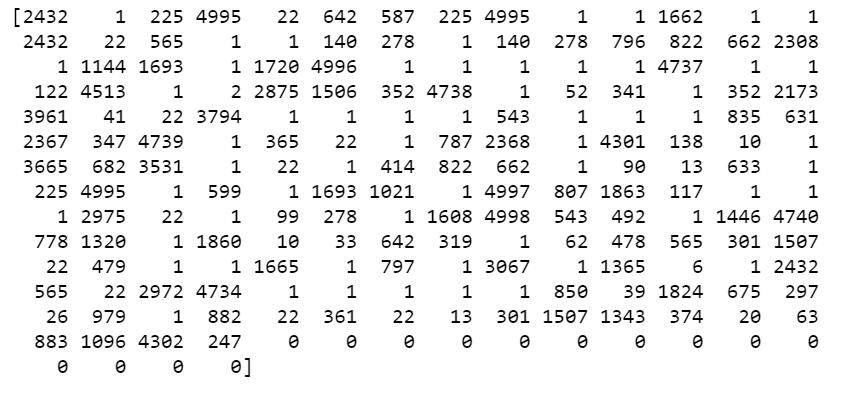" 图 2"

validation_sequences = tokenizer.texts_to_sequences(validation_articles)validation_padded = pad_sequences(validation_sequences, maxlen=max_length, padding=padding_type, truncating=trunc_type)print(len(validation_sequences))print(validation_padded.shape)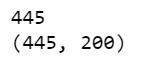label_tokenizer = Tokenizer()label_tokenizer.fit_on_texts(labels)training_label_seq = np.array(label_tokenizer.texts_to_sequences(train_labels))validation_label_seq = np.array(label_tokenizer.texts_to_sequences(validation_labels))print(training_label_seq)print(training_label_seq)print(training_label_seq)print(training_label_seq.shape)print(validation_label_seq)print(validation_label_seq)print(validation_label_seq)print(validation_label_seq.shape)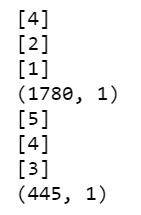reverse_word_index = dict([(value, key) for (key, value) in word_index.items()])def decode_article(text):    return ' '.join([reverse_word_index.get(i, '?') for i in text])print(decode_article(train_padded))print('---')print(train_articles)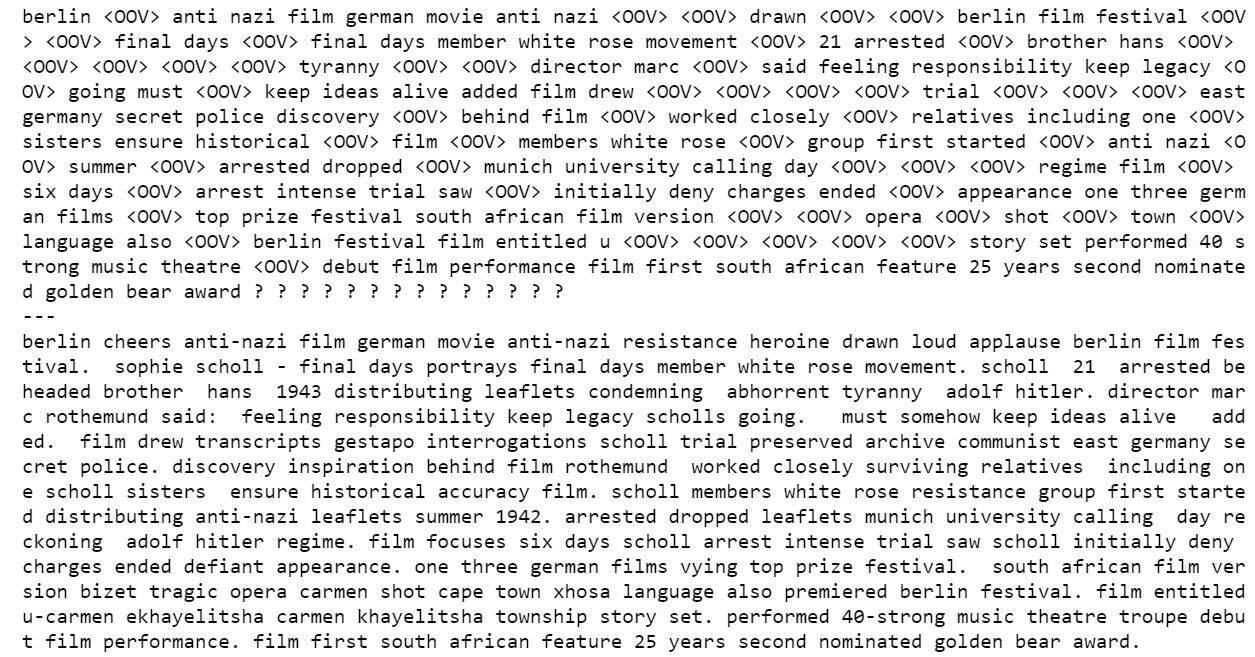“图 3”

• 我们构建了一个 tf.keras.Sequential 模型，从嵌入层开始。嵌入层为每个单词存储一个向量。调用时，它将单词索引序列转换为向量序列。经过训练后，具有相似意义的单词，通常会具有相似的向量。

• 双向包装器（Bidirectional wrapper）与 LSTM 层一起使用，它通过 LSTM 层向前和向后传播输入，然后连接输出。这有助于长短期记忆网络学习长期依赖关系。然后我们将其拟合到密集神经网络（Dense Neural Network）中进行分类。

• 我们使用 relu 代替 than 函数，因为这两个函数能够彼此很好地相互替代。

• 我们添加了 6 个单位和 softmax 激活的密集层（Dense Layer）。当我们有多个输出时，softmax 将输出层转换为概率分布。

model = tf.keras.Sequential([    # Add an Embedding layer expecting input vocab of size 5000, and output embedding dimension of size 64 we set at the top    tf.keras.layers.Embedding(vocab_size, embedding_dim),    tf.keras.layers.Bidirectional(tf.keras.layers.LSTM(embedding_dim)),#    tf.keras.layers.Bidirectional(tf.keras.layers.LSTM(32)),    # use ReLU in place of tanh function since they are very good alternatives of each other.    tf.keras.layers.Dense(embedding_dim, activation='relu'),    # Add a Dense layer with 6 units and softmax activation.    # When we have multiple outputs, softmax convert outputs layers into a probability distribution.    tf.keras.layers.Dense(6, activation='softmax')])model.summary()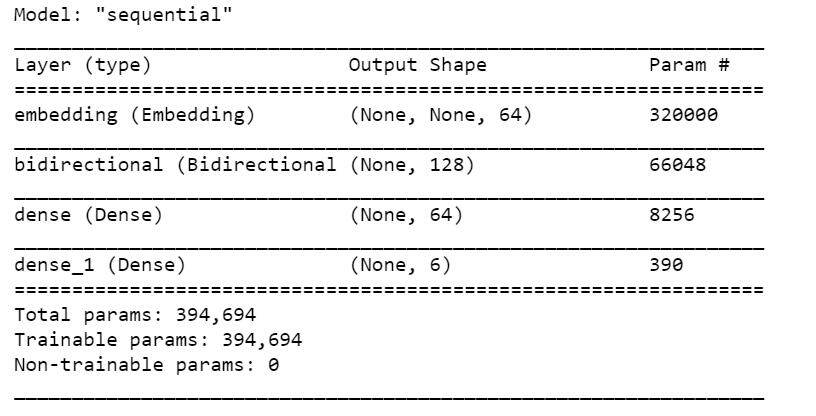“图 4”

print(set(labels))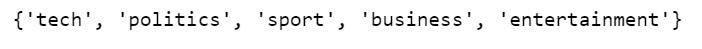sparse_categorical_crossentropy 作为损失函数，它似乎认为 0 也是一个可能的标签，而词法分析器对象是从整数 1 开始标记化，而不是整数 0。结果，尽管从未使用过 0，但最后一个密集层需要标签 0、1、2、3、4、5 的输出。

model.compile(loss='sparse_categorical_crossentropy', optimizer='adam', metrics=['accuracy'])num_epochs = 10history = model.fit(train_padded, training_label_seq, epochs=num_epochs, validation_data=(validation_padded, validation_label_seq), verbose=2)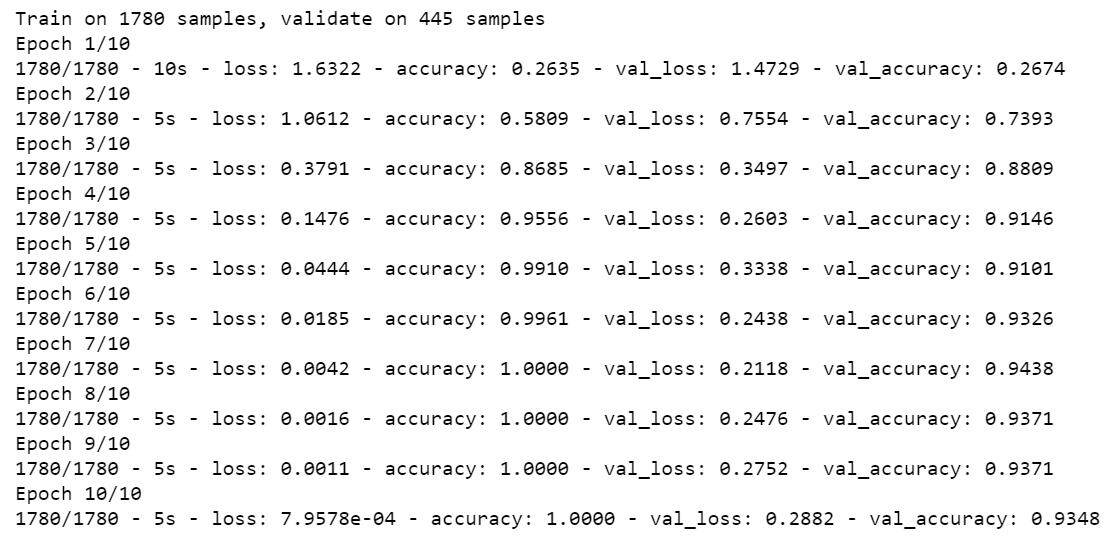“图 5”

def plot_graphs(history, string):  plt.plot(history.history[string])  plt.plot(history.history['val_'+string])  plt.xlabel("Epochs")  plt.ylabel(string)  plt.legend([string, 'val_'+string])  plt.show()plot_graphs(history, "accuracy")plot_graphs(history, "loss")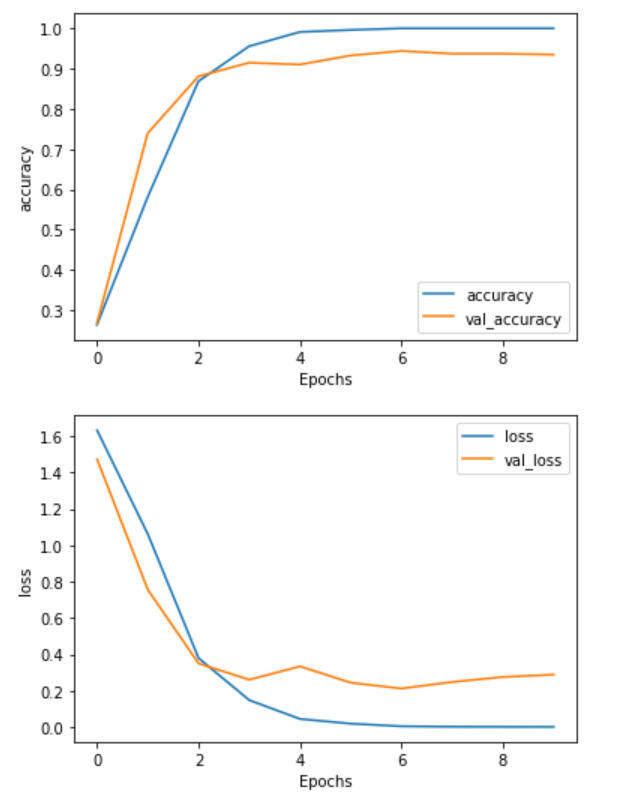#### 作者介绍：

Susan Li，是加拿大多伦多的高级数据科学家。她的理想是，每次发表文章，就改变世界。

https://towardsdatascience.com/multi-class-text-classification-with-lstm-using-tensorflow-2-0-d88627c10a35